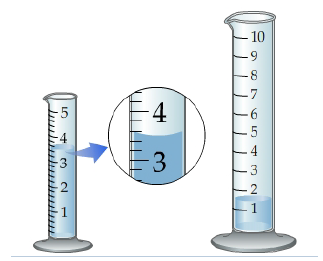Problem: Part A. How many milliliters of liquid does the smaller graduated cylinder contain?Express your answer in milliliters using the proper number of significant figures.Consider two graduated cylinders that measure in units of milliliters.

FREE Expert Solution
96% (427 ratings)Problem Details

Part A. How many milliliters of liquid does the smaller graduated cylinder contain?
Express your answer in milliliters using the proper number of significant figures.

Consider two graduated cylinders that measure in units of milliliters.